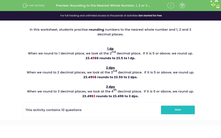# Round to the Nearest Whole Number and to 1, 2 or 3 Decimal Places

In this worksheet, students will practise rounding numbers to the nearest whole number and 1, 2 or 3 decimal places.Key stage:  KS 3

Curriculum topic:   Number

Curriculum subtopic:   Round/Approximate Numbers Appropriately

Popular topics:   Decimals worksheets, Rounding worksheets, Place Value worksheets

Difficulty level:#### Worksheet Overview

In this activity, we will practise rounding numbers to the nearest whole number and to 1, 2 and 3 decimal places.

Rounding numbers makes them easier to use, while keeping them close to the original number.

Whole Number

When we round to the nearest whole number, we look at the first decimal place. If it is 5 or above, we round up, if not we round down.

23.4966 rounds to 23 to the nearest whole number.

1 dp

When we round to 1 decimal place, we look at the second decimal place.  If it is 5 or above, we round up.

23.4966 rounds to 23.5 to 1 dp.

2 dps

When we round to 2 decimal places, we look at the third decimal place.  If it is 5 or above, we round up.

23.4966 rounds to 23.50 to 2 dps.

3 dps

When we round to 3 decimal places, we look at the fourth decimal place.  If it is 5 or above, we round up.

23.4963 rounds to 23.496 to 3 dps.

All clear!Let's try some questions now.

### What is EdPlace?

We're your National Curriculum aligned online education content provider helping each child succeed in English, maths and science from year 1 to GCSE. With an EdPlace account you’ll be able to track and measure progress, helping each child achieve their best. We build confidence and attainment by personalising each child’s learning at a level that suits them.

Get started